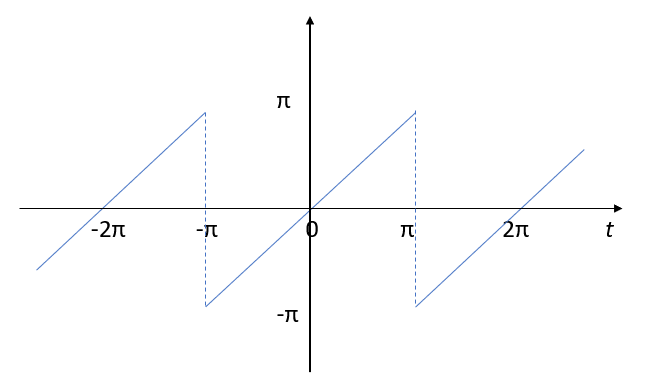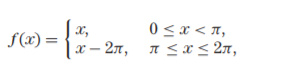# Sawtooth Function (Wave)The sawtooth function, named after it’s saw-like appearance, is a relatively simple discontinuous function, defined as f (t) = t for the initial period (from -π to π in the above image).

This periodic function then repeats (as shown by the first and last lines on the above image). The additional periods are defined by a periodic extension of f (t):
f (t + kT) = f (t).

In function notation, the sawtooth can be defined as:## Graphing the Sawtooth Function

The function is challenging to graph, but can be represented by a linear combination of sine functions.

Some mathematical software have built in functions for the sawtooth. For example, in Mathematica, the function is:
Plot[SawtoothWave[x],{x,0,1}].

## Fourier Series of the Sawtooth WaveThe sawtooth function can be represented by a Fourier series. The graph shows three terms; more are typically used.

The Fourier series representation is:The Mathematica code (Jordan, n.d.) is:
L = 10
a=2*L/Pi
one=a*Sin[Pi x/L]
two=one‐(a/2)*Sin[2 Pi x/L]
three=two + (a/3)*Sin[3 Pi x/L]
Plot[{one, two, three},{x,‐L,L}]

## Discontinuous Parts

The function has a jump discontinuity after every period. These jumps are called the function’s points of discontinuity (Edwards & Penney, 2002).

## Alternative Definition for the Sawtooth Function

The term “sawtooth function” is also sometimes also used as another name for the triangle wave function (e.g. Trott, 2004).

## References

Beerends, R. et al. (2003), Fourier and Laplace Transforms. Cambridge University Press.
Jordan, K. Fourier. Retrieved December 22, 2019 from: http://www.pitt.edu/~jordan/chem1000-s18/fourier.pdf
Spanier, J. and Oldham, K. B. An Atlas of Functions. Washington, DC: Hemisphere, p. 74, 1987.
Trott, M. (2004). The Mathematica GuideBook for Programming. New York: Springer-Verlag
Edwards, C. & Penney, D. (2007). Calculus, Early Transcendentals 7th Edition. Pearson.

CITE THIS AS:
Stephanie Glen. "Sawtooth Function (Wave)" From StatisticsHowTo.com: Elementary Statistics for the rest of us! https://www.statisticshowto.com/types-of-functions/sawtooth-function-wave/